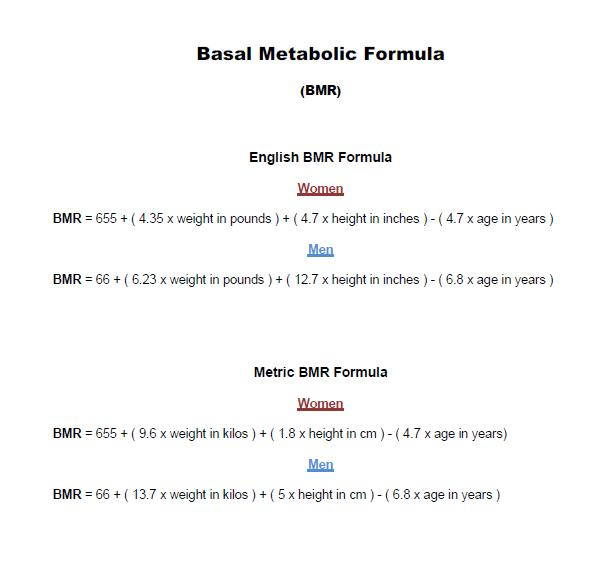# Calculating your Resting Energy ExpenditureThe Harris Benedict Equation is a formula that uses your BMR (Resting Energy Expenditure) and then applies an activity factor to determine your total daily energy expenditure (calories). The only factor omitted by the Harris Benedict Equation is lean body mass. Remember, leaner bodies need more calories than less lean ones. Therefore, this equation will be very accurate in all but the very muscular (will under-estimate calorie needs) and the very obese (will over-estimate calorie needs).

STEP 1

Plug in the appropriate numbers and solve the formula above to find your

BMR (Basal Metabolic Rate)

BMR formula (Pdf Chart)

English BMR Formula

Women

BMR = 655 + ( 4.35 x weight in pounds ) + ( 4.7 x height in inches ) - ( 4.7 x age in years )

Men

BMR = 66 + ( 6.23 x weight in pounds ) + ( 12.7 x height in inches ) - ( 6.8 x age in years )

Metric BMR Formula

Women

BMR = 655 + ( 9.6 x weight in kilos ) + ( 1.8 x height in cm ) - ( 4.7 x age in years)

Men

BMR = 66 + ( 13.7 x weight in kilos ) + ( 5 x height in cm ) - ( 6.8 x age in years )

STEP 2

After you determine the BMR (Basal Metabolic Rate) choose the appropriate

category from the chart. Use the Harris Benedict Formula To determine your total daily calorie needs, multiply your BMR by the appropriate activity factor, as follows:

1. If you are sedentary (little or no exercise): Calorie-Calculation = BMR x 1.2

2. If you are lightly active (light exercise/sports 1-3 days/week): Calorie-Calculation =

BMR x 1.375

3. If you are moderately active (moderate exercise/sports 3-5 days/week): Calorie-

Calculation = BMR x 1.55

4.If you are very active (hard exercise/sports 6-7 days a week): Calorie-Calculation =

BMR x 1.725

5.If you are extra active (very hard exercise/sports & physical job or 2x training):

Calorie-Calculation = BMR x 1.9

STEP 3

After you choose the appropriate category, multiply your BMR times the proper

number. The answer will give you an estimate of your calories burned at rest.